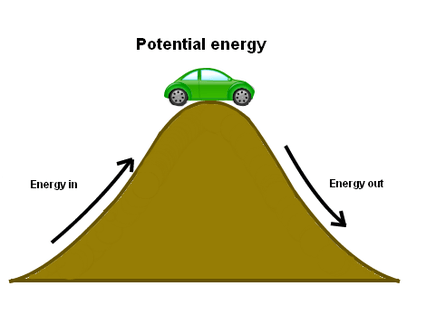# Potential Energy Calculator

To calculate PE= mgh by using this Potential Energy Calculator., Enter the values in the given input boxes and hit the "calculate" button

Formula PE = m * g * h## Formula

Potential energy formula & equation

Mass :
m = PE/g*h

Acceleration of Gravity :
g = PE/m*h

Height :
h = PE/m*g

Potential Energy :
PE = m x g x h

Where,
m = Mass,
g = Acceleration of Gravity
h = Height.
Gravitational Acceleration of the earth is 9.8 m/sec2.Our Potential Energy Calculator uses gravitational potential energy formula. You can also calculate Mass, Acceleration of Gravity and Height. Potential Energy refers to the energy of a body or a system with respect to the position of the body or the arrangement of the particles of the system.

Potential energy refers to the stored energy of position possessed by an object. The Potential Energy is denoted by the symbol ‘PE’. Potential energy has the ability to convert into other forms of energy like kinetic energy. The Potential Energy is measured in joule.

The existence of the potential energy is seen when a force acts upon an object that tends to restore it to a lower energy configuration. The term "potential energy" was coined by the 19th-century Scottish engineer and physicist William Rankine. In science, the potential energy is expressed as  PE, U, or V.

Example:
Calculate the potential energy for the given details.
Mass (m) = 10 kg
Acceleration of Gravity (g) = 2 m/s2
Height (h) = 20 m

Solution:
Apply Formula:
PE = m x g x h
PE = 10*2*20
PE = 400 Joule
Potential Energy (P.E) = 400 J

1. "Potential Energy". Jersey.Uoregon.Edu,
2. Potential Energy - Knowledge Bank - Solar Schools.
3. Potential energy | Definition, Examples, & Facts.britannica.com
4. What is Potential Energy? Gravitational Potential Energy,physicsclassroom.com
5. Davis, M. (2020). What Is Potential Energy? livescience.com